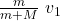Question

n the railroad accident, a boxcar weighting 200 kN and traveling at 3 m/s on horizontal track slams into a stationary caboose weighting 400 kN. The collision connects the caboose to the car, and then both move together and you have found the final velocity. Apparently, initial kinetic energy of the system changes (in part, because of friction present). How much energy (in kJ) is transferred from kinetic energy to other forms of energy (e..g., thermal) in the collision

1.ΔK = -6 10⁴ J

Explanation:

This is a crash problem, let’s start by defining a system formed by the two trucks, so that the forces during the crash have been internal and the moment is preserved

initial instant. Before the crash

p₀ = m v₁ + M 0

final instant. Right after the crash

p_f = (m + M) v

p₀ = p_f

mv₁ = (m + M) v

v =we substitute

v =3

v = 1.0 m / s

having the initial and final velocities, let’s find the kinetic energy

K₀ = ½ m v₁² + 0

K₀ = ½ 20 10³ 3²

K₀ = 9 10⁴ J

K_f = ½ (m + M) v²

K_f = ½ (20 +40) 10³  1²

K_f = 3 10⁴ J

the change in energy is

ΔK = K_f – K₀

ΔK = (3 – 9) 10⁴

ΔK = -6 10⁴ J

The negative sign indicates that the energy is ranked in another type of energy